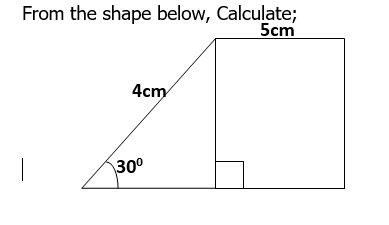### Mathematics JSS3 Second Term Final Assessment

Assessment

1. Solve 2x-y=6 and x+y=6. Using substitution method

1. Y = 2 , x = 4
2. Y = 4, x = 2
3. Y = -2, x = -4
4. Y = -4, x = -2

2. Solve 2x-y=6 and x + y=6. Using elimination method

1.  Y = 2 , x = 4
2. Y = 4, x = 2
3. Y = -2, x = -4
4. Y = -4, x = -2

3. State the Area and Perimeter of the following

1. Prizm
2. Cuboid
3. Pyramid
4. Sphere
5. Cube3. The Area

1. 11.46cm2
2. 12.46cm2
3. 13.46cm2
4. 14.46cm2

4. The Perimeter

1. 24.4cm
2. 25.4cm
3. View More